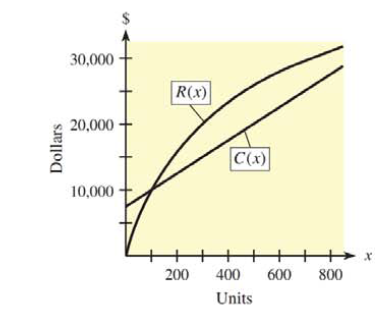Chapter 9.9, Problem 30EMathematical Applications for the ...

12th Edition
Ronald J. Harshbarger + 1 other
ISBN: 9781337625340

Solutions

Chapter
SectionMathematical Applications for the ...

12th Edition
Ronald J. Harshbarger + 1 other
ISBN: 9781337625340
Textbook Problem

In each of Problems 29 and 30, the graphs of a company’s total revenue function and total cost function are shown. For each problem, use the graph to answer the following questions.(a) From the sale of 100 items, 400 items, and 700 items, rank from smallest to largest the amount of profit received. Explain your choices and note whether any of these scenarios results in a loss.(b) From the sale of the 101st item, the 401st item, and the 701st item, rank from smallest to largest the amount of profit received. Explain your choices, and note whether any of these scenarios results in a loss.(a)

To determine

The rank order from smallest to largest profit for the sale of 100 items, 400 items and 700 from the graph of a company’s total revenue and total cost function.

Explanation

Given Information:

The graph of the revenue function and total cost function of the company is shown below:

Explanation:

Consider the sales of the company are 100 units, 400 units and 700 units.

The profit (P) of the sales is the difference of the revenue (R) generated by the company through sale and the cost (C) of the units produced,

P=RC

The graph of the revenue function and total cost function of the company is shown below:

From the graph, when the number of units sell is 100, the corresponding values for revenue and the cost are approximate 10,000 and 10,000 respectively.

Substitute 10,000 for R and 10,000 for P in P=RC to get the profit,

P=10,00010,000=0

From the graph, when the number of units sell is 400, the corresponding values for revenue and the cost are approximate 22,000 and 15,000 respectively

(b)

To determine

The rank order from smallest to largest profit for the sale of 100st items, 400st items and 700st items from the graph of a company’s total revenue and total cost function.

Still sussing out bartleby?

Check out a sample textbook solution.

See a sample solution

The Solution to Your Study Problems

Bartleby provides explanations to thousands of textbook problems written by our experts, many with advanced degrees!

Get Started

Find the distance between the points (-2, 1) and (2, 4).

Calculus: An Applied Approach (MindTap Course List)

Draw a polygon for the distribution of scores shown in the following table. X f 6 2 5 5 4 3 3 2 2 1

Essentials of Statistics for The Behavioral Sciences (MindTap Course List)

Convert the expressions in Exercises 6584 to power form. 1(x2+1)334(x2+1)3

Finite Mathematics and Applied Calculus (MindTap Course List)

Evaluate the integral. x1+x3dx

Calculus (MindTap Course List)

Subtract the following numbers. 19.

Contemporary Mathematics for Business & Consumers

If n(A)=10, n(AB)=15 and n(B)=8, then what is n(AB)?

Finite Mathematics for the Managerial, Life, and Social Sciences

∫ sin 3x cos 6x dx =

Study Guide for Stewart's Single Variable Calculus: Early Transcendentals, 8th